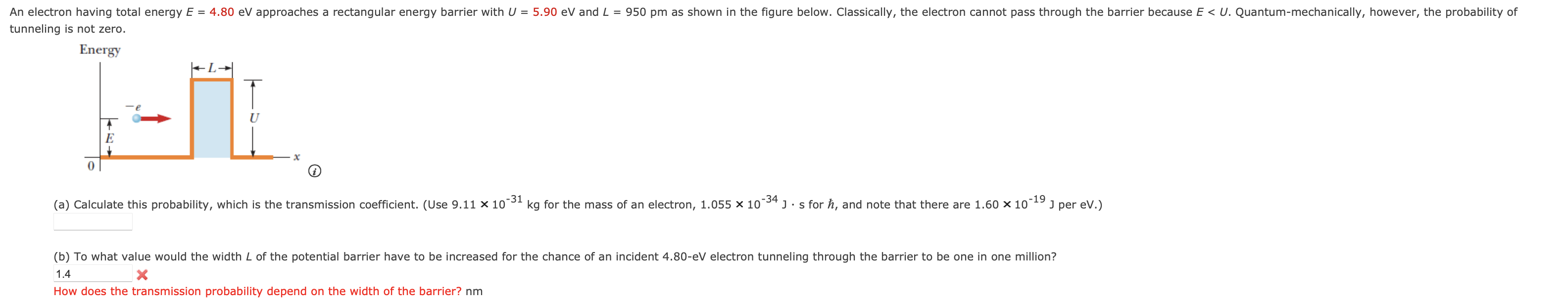Home / Expert Answers / Physics / 1-how-does-the-transmission-probability-depend-on-the-width-of-the-barrier-nm-pa910

# (Solved): 1 How does the transmission probability depend on the width of the barrier? nm ...1 How does the transmission probability depend on the width of the barrier?

We have an Answer from Expert

Given data,

Total energy, E = 4.80 eV

Energy barrier, E = 5.90 eV

Length, L = 950 pm = 950 x 10-12 m

We have an Answer from Expert